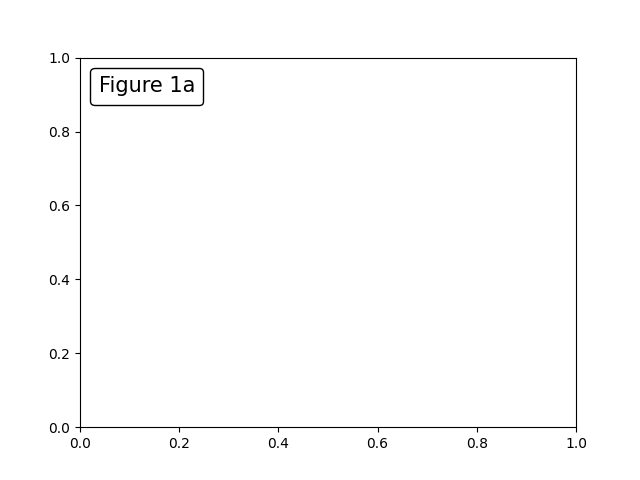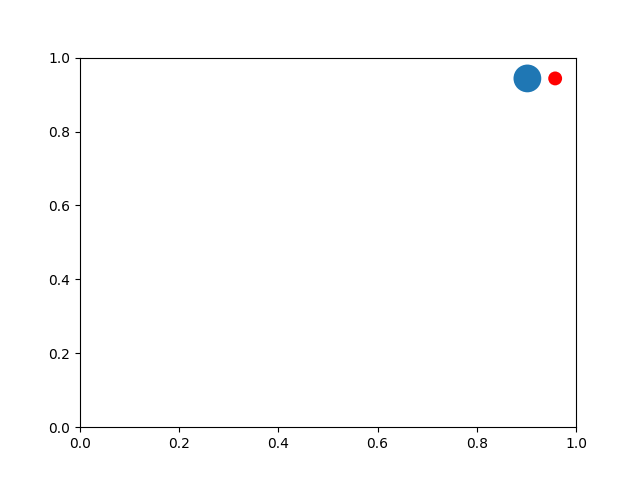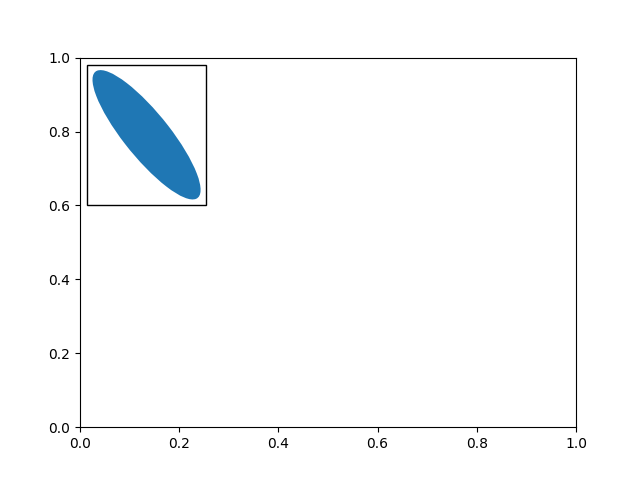# Annotations#

Annotating text with Matplotlib.

```from matplotlib import pyplot as plt
```

## Basic annotation#

The uses of the basic `text()` will place text at an arbitrary position on the Axes. A common use case of text is to annotate some feature of the plot, and the `annotate()` method provides helper functionality to make annotations easy. In an annotation, there are two points to consider: the location being annotated represented by the argument xy and the location of the text xytext. Both of these arguments are `(x, y)` tuples.

In this example, both the xy (arrow tip) and xytext locations (text location) are in data coordinates. There are a variety of other coordinate systems one can choose -- you can specify the coordinate system of xy and xytext with one of the following strings for xycoords and textcoords (default is 'data')

argument

coordinate system

'figure points'

points from the lower left corner of the figure

'figure pixels'

pixels from the lower left corner of the figure

'figure fraction'

(0, 0) is lower left of figure and (1, 1) is upper right

'axes points'

points from lower left corner of axes

'axes pixels'

pixels from lower left corner of axes

'axes fraction'

(0, 0) is lower left of axes and (1, 1) is upper right

'data'

use the axes data coordinate system

For example to place the text coordinates in fractional axes coordinates, one could do:

```ax.annotate('local max', xy=(3, 1),  xycoords='data',
xytext=(0.8, 0.95), textcoords='axes fraction',
arrowprops=dict(facecolor='black', shrink=0.05),
horizontalalignment='right', verticalalignment='top',
)
```

For physical coordinate systems (points or pixels) the origin is the bottom-left of the figure or axes.

Optionally, you can enable drawing of an arrow from the text to the annotated point by giving a dictionary of arrow properties in the optional keyword argument arrowprops.

arrowprops key

description

width

the width of the arrow in points

frac

the fraction of the arrow length occupied by the head

the width of the base of the arrow head in points

shrink

move the tip and base some percent away from the annotated point and text

**kwargs

any key for `matplotlib.patches.Polygon`, e.g., `facecolor`

In the example below, the xy point is in native coordinates (xycoords defaults to 'data'). For a polar axes, this is in (theta, radius) space. The text in this example is placed in the fractional figure coordinate system. `matplotlib.text.Text` keyword arguments like horizontalalignment, verticalalignment and fontsize are passed from `annotate` to the `Text` instance.

For more on all the wild and wonderful things you can do with annotations, including fancy arrows, see Advanced Annotations and Annotating Plots.

Do not proceed unless you have already read Basic annotation, `text()` and `annotate()`!

### Annotating with Text with Box#

`text` takes a bbox keyword argument, which draws a box around the text:

```t = ax.text(
0, 0, "Direction", ha="center", va="center", rotation=45, size=15,
```

The patch object associated with the text can be accessed by:

```bb = t.get_bbox_patch()
```

The return value is a `FancyBboxPatch`; patch properties (facecolor, edgewidth, etc.) can be accessed and modified as usual. `FancyBboxPatch.set_boxstyle` sets the box shape:

```bb.set_boxstyle("rarrow", pad=0.6)
```

The arguments are the name of the box style with its attributes as keyword arguments. Currently, following box styles are implemented.

Class

Name

Attrs

Circle

`circle`

DArrow

`darrow`

LArrow

`larrow`

RArrow

`rarrow`

Round

`round`

Round4

`round4`

Roundtooth

`roundtooth`

Sawtooth

`sawtooth`

Square

`square`

Note that the attribute arguments can be specified within the style name with separating comma (this form can be used as "boxstyle" value of bbox argument when initializing the text instance)

```bb.set_boxstyle("rarrow,pad=0.6")
```

### Annotating with Arrow#

`annotate` draws an arrow connecting two points in an Axes:

```ax.annotate("Annotation",
xy=(x1, y1), xycoords='data',
xytext=(x2, y2), textcoords='offset points',
)
```

This annotates a point at xy in the given coordinate (xycoords) with the text at xytext given in textcoords. Often, the annotated point is specified in the data coordinate and the annotating text in offset points. See `annotate` for available coordinate systems.

An arrow connecting xy to xytext can be optionally drawn by specifying the arrowprops argument. To draw only an arrow, use empty string as the first argument.

```ax.annotate("",
xy=(0.2, 0.2), xycoords='data',
xytext=(0.8, 0.8), textcoords='data',
arrowprops=dict(arrowstyle="->",
connectionstyle="arc3"),
)
```

The arrow is drawn as follows:

1. A path connecting the two points is created, as specified by the connectionstyle parameter.

2. The path is clipped to avoid patches patchA and patchB, if these are set.

3. The path is further shrunk by shrinkA and shrinkB (in pixels).

4. The path is transmuted to an arrow patch, as specified by the arrowstyle parameter.

The creation of the connecting path between two points is controlled by `connectionstyle` key and the following styles are available.

Name

Attrs

`angle`

`angle3`

angleA=90,angleB=0

`arc`

`arc3`

`bar`

armA=0.0,armB=0.0,fraction=0.3,angle=None

Note that "3" in `angle3` and `arc3` is meant to indicate that the resulting path is a quadratic spline segment (three control points). As will be discussed below, some arrow style options can only be used when the connecting path is a quadratic spline.

The behavior of each connection style is (limitedly) demonstrated in the example below. (Warning: The behavior of the `bar` style is currently not well defined, it may be changed in the future).

The connecting path (after clipping and shrinking) is then mutated to an arrow patch, according to the given `arrowstyle`.

Name

Attrs

`-`

None

`->`

`-[`

widthB=1.0,lengthB=0.2,angleB=None

`|-|`

widthA=1.0,widthB=1.0

`-|>`

`<-`

`<->`

`<|-`

`<|-|>`

`fancy`

`simple`

`wedge`

tail_width=0.3,shrink_factor=0.5

Some arrowstyles only work with connection styles that generate a quadratic-spline segment. They are `fancy`, `simple`, and `wedge`. For these arrow styles, you must use the "angle3" or "arc3" connection style.

If the annotation string is given, the patchA is set to the bbox patch of the text by default.

As with `text`, a box around the text can be drawn using the bbox argument.

By default, the starting point is set to the center of the text extent. This can be adjusted with `relpos` key value. The values are normalized to the extent of the text. For example, (0, 0) means lower-left corner and (1, 1) means top-right.

### Placing Artist at anchored Axes locations#

There are classes of artists that can be placed at an anchored location in the Axes. A common example is the legend. This type of artist can be created by using the `OffsetBox` class. A few predefined classes are available in `matplotlib.offsetbox` and in `mpl_toolkits.axes_grid1.anchored_artists`.

```from matplotlib.offsetbox import AnchoredText

fig, ax = plt.subplots()
at = AnchoredText(
"Figure 1a", prop=dict(size=15), frameon=True, loc='upper left')
```The loc keyword has same meaning as in the legend command.

A simple application is when the size of the artist (or collection of artists) is known in pixel size during the time of creation. For example, If you want to draw a circle with fixed size of 20 pixel x 20 pixel (radius = 10 pixel), you can utilize `AnchoredDrawingArea`. The instance is created with a size of the drawing area (in pixels), and arbitrary artists can added to the drawing area. Note that the extents of the artists that are added to the drawing area are not related to the placement of the drawing area itself. Only the initial size matters.

The artists that are added to the drawing area should not have a transform set (it will be overridden) and the dimensions of those artists are interpreted as a pixel coordinate, i.e., the radius of the circles in above example are 10 pixels and 5 pixels, respectively.

```from matplotlib.patches import Circle
from mpl_toolkits.axes_grid1.anchored_artists import AnchoredDrawingArea

fig, ax = plt.subplots()
ada = AnchoredDrawingArea(40, 20, 0, 0,
p1 = Circle((10, 10), 10)
p2 = Circle((30, 10), 5, fc="r")
```Sometimes, you want your artists to scale with the data coordinate (or coordinates other than canvas pixels). You can use `AnchoredAuxTransformBox` class. This is similar to `AnchoredDrawingArea` except that the extent of the artist is determined during the drawing time respecting the specified transform.

The ellipse in the example below will have width and height corresponding to 0.1 and 0.4 in data coordinates and will be automatically scaled when the view limits of the axes change.

```from matplotlib.patches import Ellipse
from mpl_toolkits.axes_grid1.anchored_artists import AnchoredAuxTransformBox

fig, ax = plt.subplots()
box = AnchoredAuxTransformBox(ax.transData, loc='upper left')
el = Ellipse((0, 0), width=0.1, height=0.4, angle=30)  # in data coordinates!
```As in the legend, the bbox_to_anchor argument can be set. Using the HPacker and VPacker, you can have an arrangement(?) of artist as in the legend (as a matter of fact, this is how the legend is created).

Note that unlike the legend, the `bbox_transform` is set to IdentityTransform by default.

### Coordinate systems for Annotations#

Matplotlib Annotations support several types of coordinates. Some are described in Basic annotation; more advanced options are

1. A `Transform` instance. For example,

```ax.annotate("Test", xy=(0.5, 0.5), xycoords=ax.transAxes)
```

is identical to

```ax.annotate("Test", xy=(0.5, 0.5), xycoords="axes fraction")
```

This allows annotating a point in another axes:

```fig, (ax1, ax2) = plt.subplots(1, 2)
ax2.annotate("Test", xy=(0.5, 0.5), xycoords=ax1.transData,
xytext=(0.5, 0.5), textcoords=ax2.transData,
arrowprops=dict(arrowstyle="->"))
```
2. An `Artist` instance. The xy value (or xytext) is interpreted as a fractional coordinate of the bbox (return value of get_window_extent) of the artist:

```an1 = ax.annotate("Test 1", xy=(0.5, 0.5), xycoords="data",
va="center", ha="center",
bbox=dict(boxstyle="round", fc="w"))
an2 = ax.annotate("Test 2", xy=(1, 0.5), xycoords=an1,  # (1, 0.5) of the an1's bbox
xytext=(30, 0), textcoords="offset points",
va="center", ha="left",
bbox=dict(boxstyle="round", fc="w"),
arrowprops=dict(arrowstyle="->"))
```

Note that you must ensure that the extent of the coordinate artist (an1 in above example) is determined before an2 gets drawn. Usually, this means that an2 needs to be drawn after an1.

3. A callable object that takes the renderer instance as single argument, and returns either a `Transform` or a `BboxBase`. The return value is then handled as in (1), for transforms, or in (2), for bboxes. For example,

```an2 = ax.annotate("Test 2", xy=(1, 0.5), xycoords=an1,
xytext=(30, 0), textcoords="offset points")
```

is identical to:

```an2 = ax.annotate("Test 2", xy=(1, 0.5), xycoords=an1.get_window_extent,
xytext=(30, 0), textcoords="offset points")
```
4. A pair of coordinate specifications -- the first for the x-coordinate, and the second is for the y-coordinate; e.g.

```annotate("Test", xy=(0.5, 1), xycoords=("data", "axes fraction"))
```

Here, 0.5 is in data coordinates, and 1 is in normalized axes coordinates. Each of the coordinate specifications can also be an artist or a transform. For example,

5. Sometimes, you want your annotation with some "offset points", not from the annotated point but from some other point. `text.OffsetFrom` is a helper for such cases.

You may take a look at this example Annotating Plots.

### Using ConnectionPatch#

`ConnectionPatch` is like an annotation without text. While `annotate` is sufficient in most situations, `ConnectionPatch` is useful when you want to connect points in different axes.

```from matplotlib.patches import ConnectionPatch
xy = (0.2, 0.2)
con = ConnectionPatch(xyA=xy, coordsA=ax1.transData,
xyB=xy, coordsB=ax2.transData)
```

The above code connects point xy in the data coordinates of `ax1` to point xy in the data coordinates of `ax2`. Here is a simple example.

Here, we added the `ConnectionPatch` to the figure (with `add_artist`) rather than to either axes: this ensures that it is drawn on top of both axes, and is also necessary if using constrained_layout for positioning the axes.

### Zoom effect between Axes#

`mpl_toolkits.axes_grid1.inset_locator` defines some patch classes useful for interconnecting two axes. Understanding the code requires some knowledge of Matplotlib's transform system.

### Define Custom BoxStyle#

You can use a custom box style. The value for the `boxstyle` can be a callable object in the following forms.:

```def __call__(self, x0, y0, width, height, mutation_size,
aspect_ratio=1.):
'''
Given the location and size of the box, return the path of
the box around it.

- *x0*, *y0*, *width*, *height* : location and size of the box
- *mutation_size* : a reference scale for the mutation.
- *aspect_ratio* : aspect-ratio for the mutation.
'''
path = ...
return path
```

Here is a complete example.

Similarly, you can define a custom ConnectionStyle and a custom ArrowStyle. See the source code of `lib/matplotlib/patches.py` and check how each style class is defined.

Gallery generated by Sphinx-Gallery